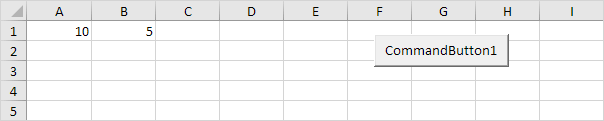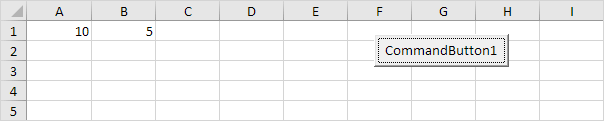# Swap Values

This example teaches you how to swap two values in Excel VBA. You will often need this structure in more complicated programs as we will see later.

Situation:Place a command button on your worksheet and add the following code lines:

1. First, we declare a variable called temp of type Double.

Dim temp As Double

2. We initialize the variable temp with the value of cell A1.

temp = Range("A1").Value

3. Now we can safely write the value of cell B1 to cell A1 (we have stored the value of cell A1 to temp so we will not lose it).

Range("A1").Value = Range("B1").Value

4. Finally, we write the value of cell A1 (written to temp) to cell B1.

Range("B1").Value = temp

5. Click the command button two times.

Result:Go to Next Chapter: MsgBox